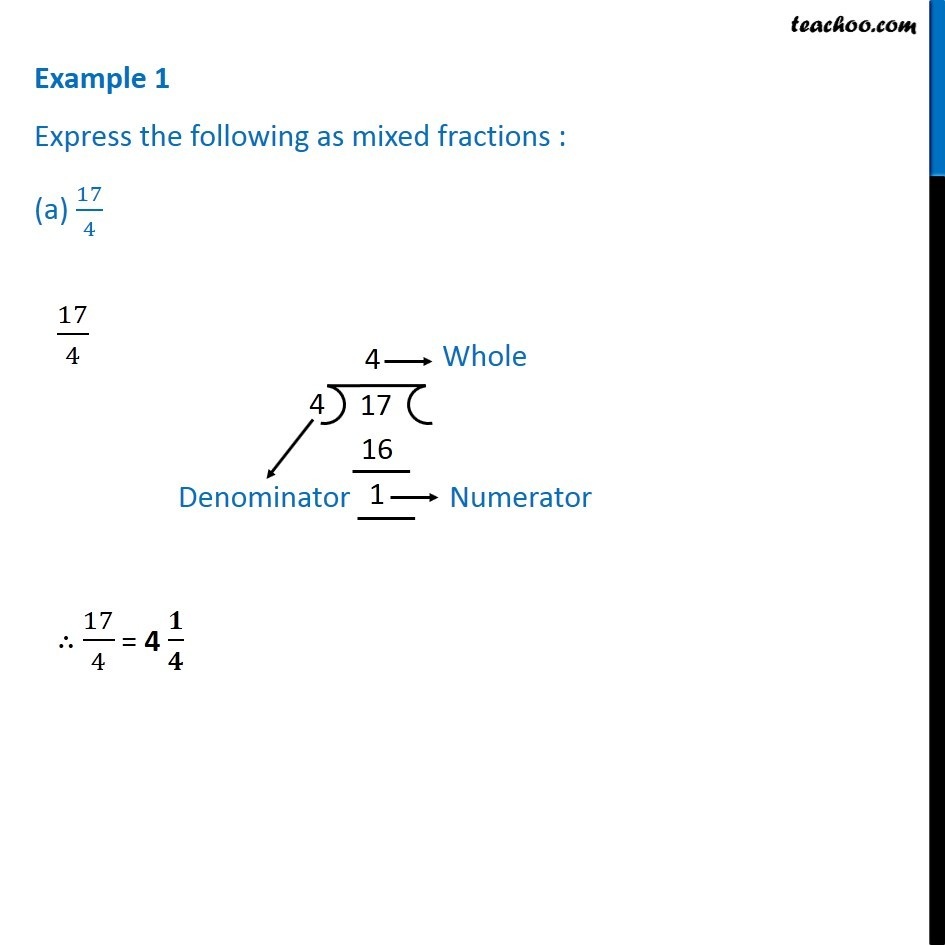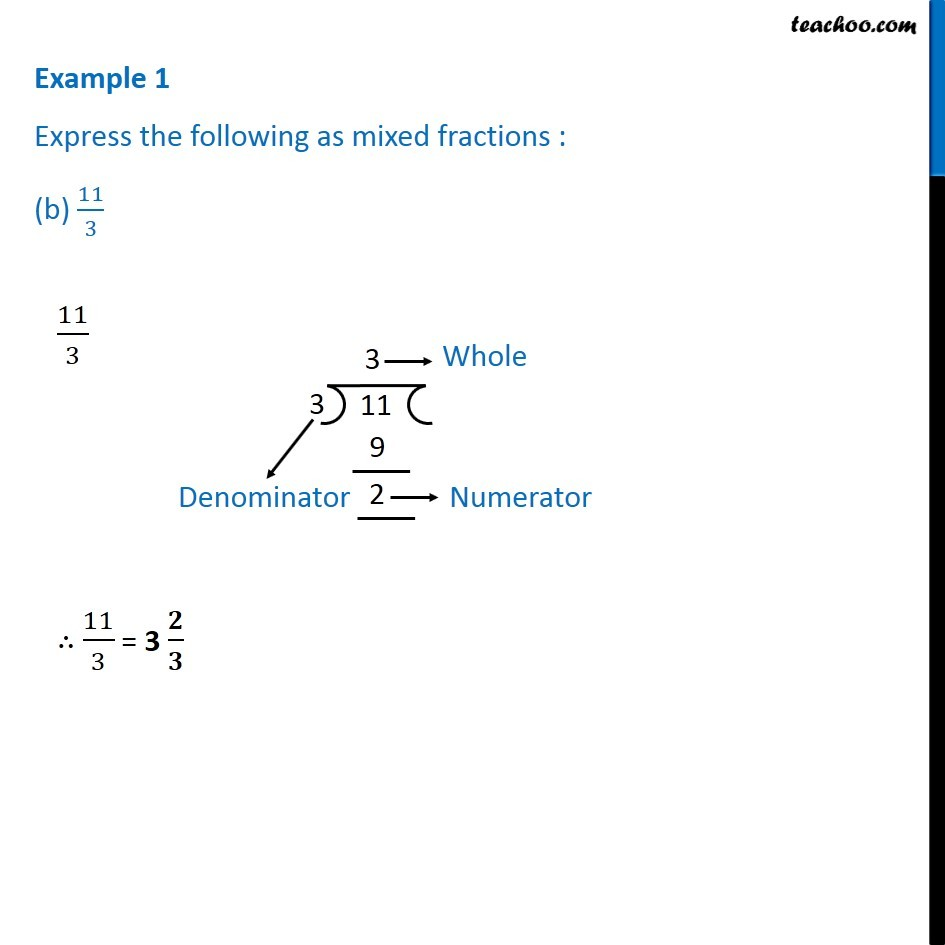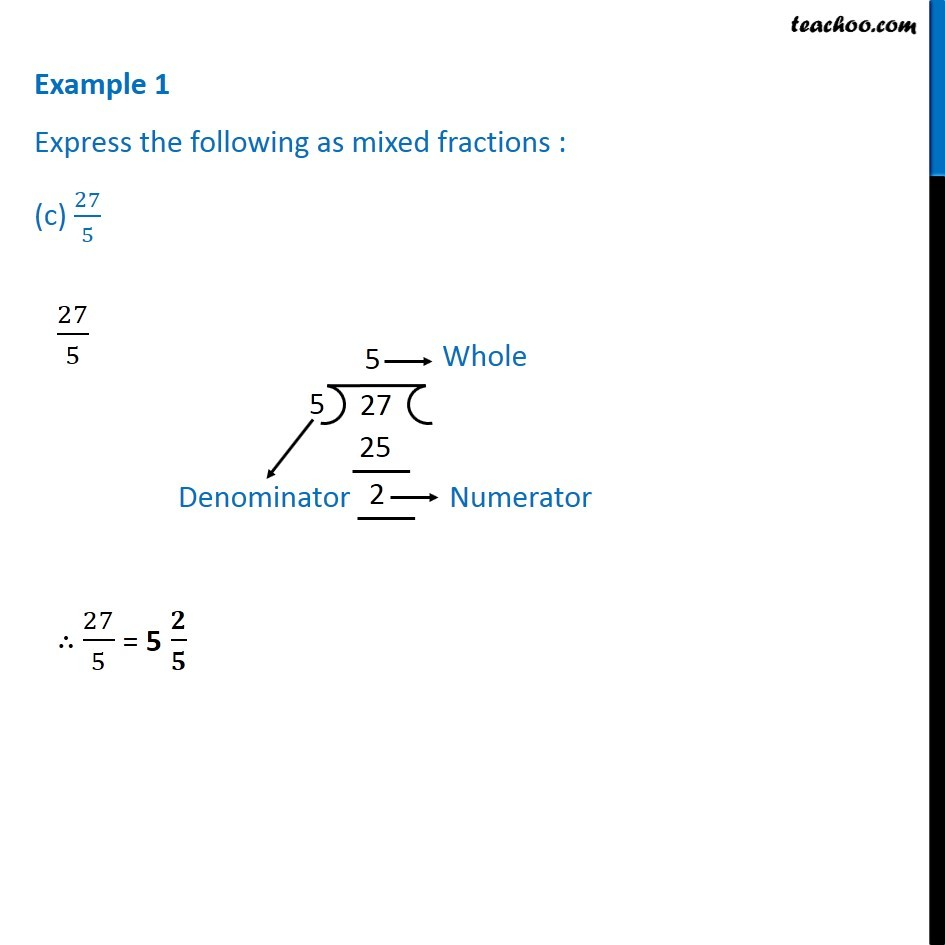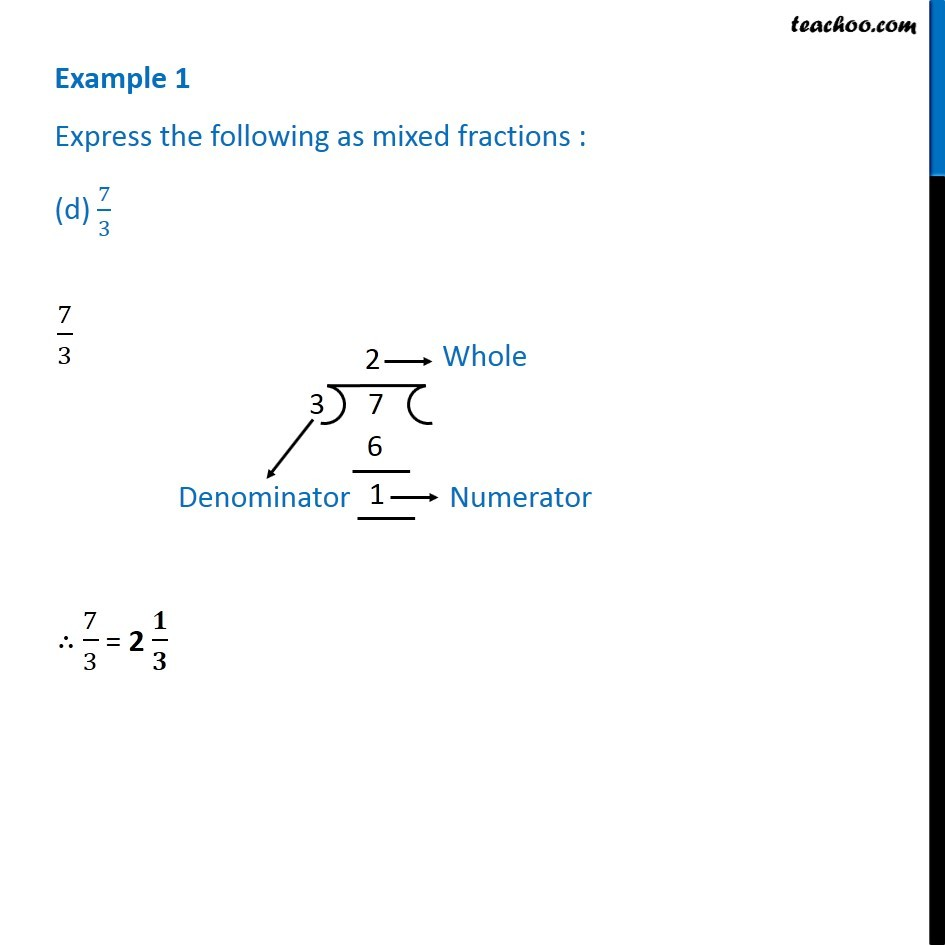Subscribe to our Youtube Channel - https://you.tube/teachoo

1. Chapter 7 Class 6 Fractions
2. Serial order wise
3. Examples

Transcript

Example 1 Express the following as mixed fractions : (a) 17/4 17/4 17/4 = 4 / 11/3 11/3 = 3 / Example 1 Express the following as mixed fractions : (c) 27/5 27/5 27/5 = 5 / Example 1 Express the following as mixed fractions : (d) 7/3 7/3 7/3 = 2 /

Examples

Chapter 7 Class 6 Fractions
Serial order wise